Glencoe Algebra 1: Glencoe Algebra 1 Flashcards

Set Details Share
created 2 months ago by sjohn4158
5 views
updated 2 months ago by sjohn4158
Subjects:
math
Page to share:
Embed this setcancel
COPY
code changes based on your size selection
Size:
X
1

POLYNOMIAL

A monomial or sum of monomials

2

MONOMIAL

a number, a variable, a product of a number and one or more variables

3

BINOMIAL

An algebraic expression that has two terms

4

TRINOMIAL

An algebraic expression that has two terms

5

MINIMUM (OPEN UPWARDS)6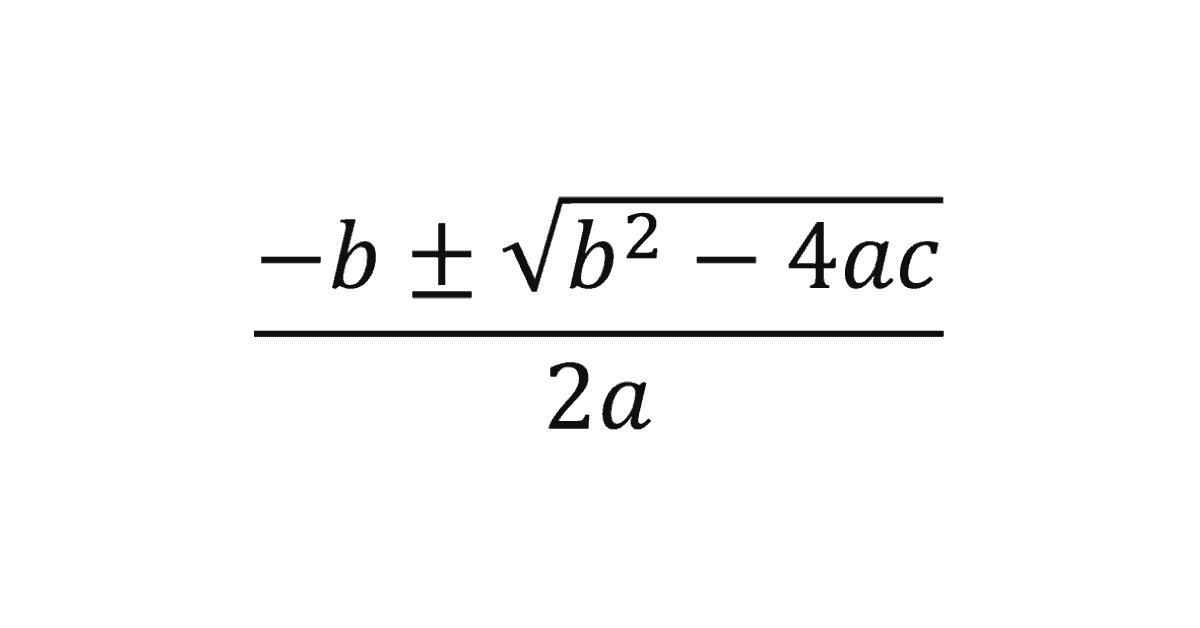7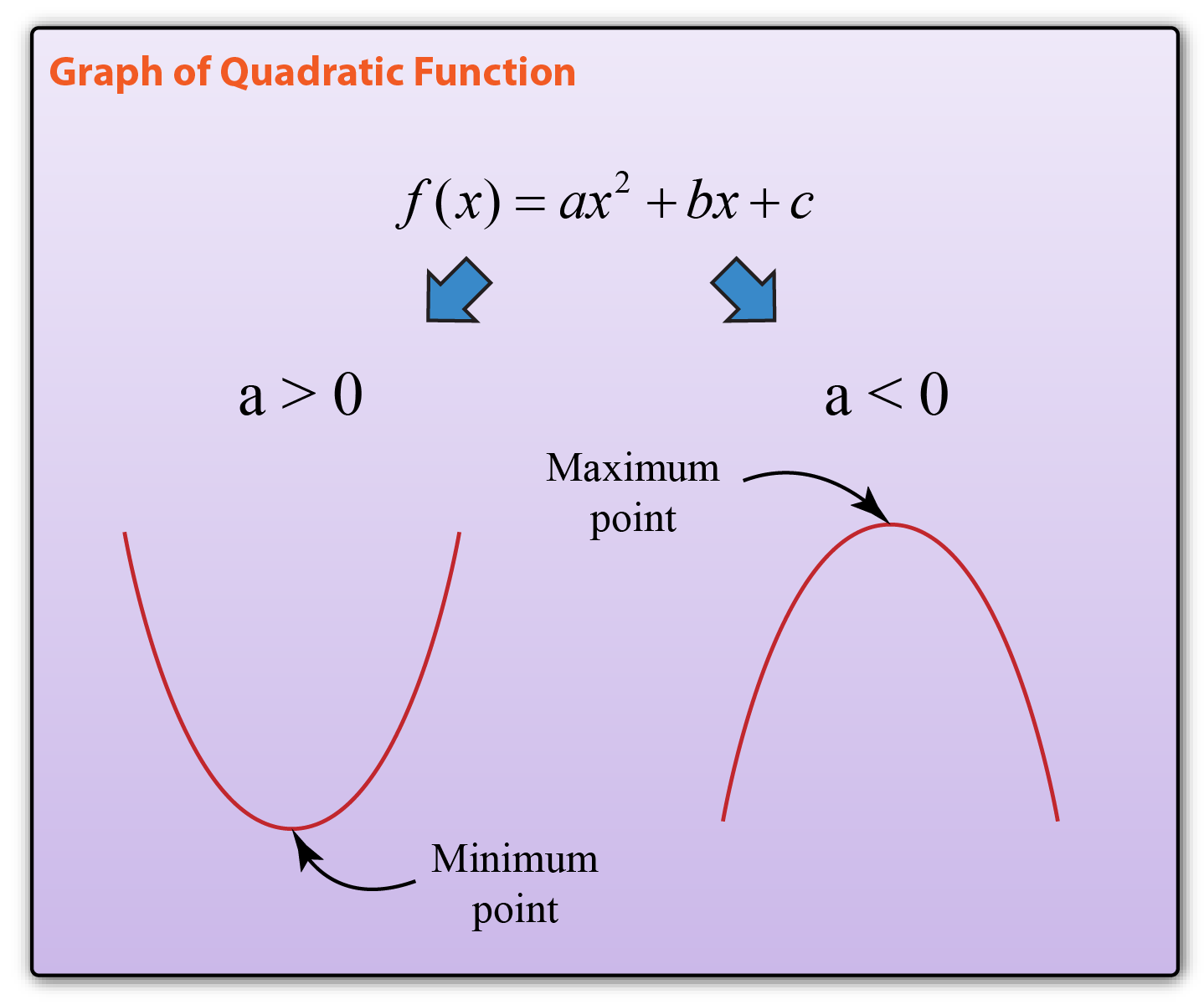8

OPENS DOWNWARD (MAXIMUM)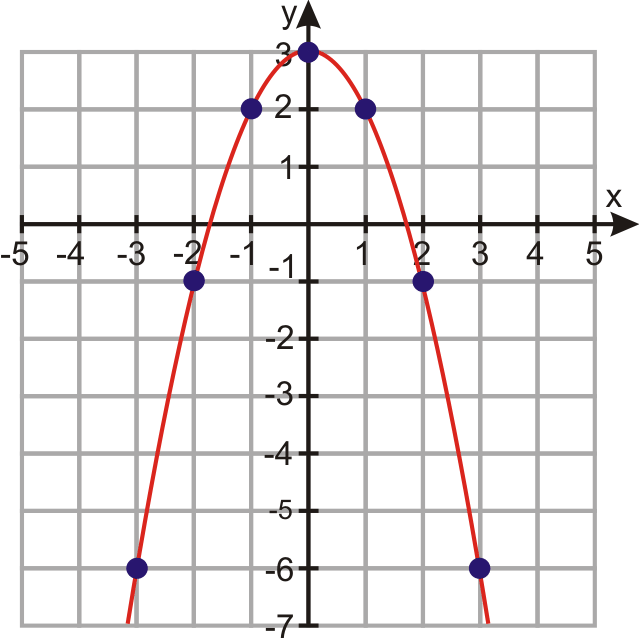9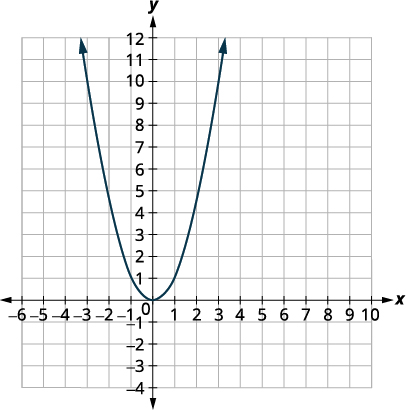Equation y= x2 Minimum

Vertex (0 ,0)

Axis of Symmetry x=0

10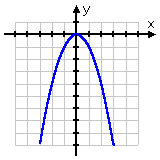Equation y= -x2 Maximum

Vertex (0,0)

Axis of Symmetry x=0

11

DIFFERENCE OF SQUARES

a2 -b2 = (a+b)(a-b)

12

PERFECT SQUARE TRINOMIAL

a2 +2ab+b2= (a+b)2

a2 -2ab+b2 = (a-b)2